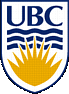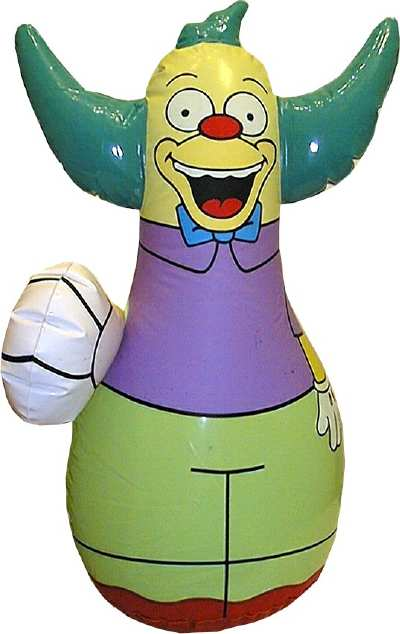UBC Physics - Air Pressure Demonstration

main

theory

lesson plan

kinetic gas simulator

hovercraft design

acknowledgements

Theory

The presentation deals with three major physical concepts: pressure, kinetic gas theory, and pressure equilibrium.

Pressure

Pressure is defined as force per unit area. It is measured in Pascals (Newtons / m^2).

Pressure = Force / Area

There are some important things to note:

• with a constant pressure, a larger area will require a larger force and vice versa
• with a constant force, a larger area will result in a smaller pressure and vice versa

In the case of a hovercraft, the large area of the base allows for a greater force to be applied with a resonable pressure

Kinetic Gas Theory

The kinetic gas theory states that gas molecules act as free particles in a container, and the speed of the particles is determined by their temperature; higher temperatures equate to faster particles. These particles bounce around colliding with one another as well as the walls of the container. The net effect of these collisions with the walls of the container exude a force over the surface area of the container, and thus a net pressure can be measured.The net pressure of the gas on the walls of the container can be described by the ideal gas law:

P = NkT/V

N = Number of Particles
T = Temperature of the Gas
V = Volume of the Container
k = 1.38 x 10-24 J/K

Note that the pressure of the gas on the walls of the container are directly proportional to the number of particles and the temperature (speed) of those particles, but inversely proportional to the volume of the container.

Pressure Equilibrium

In the atmosphere, systems will change to equalize pressure on both sides of a surface. In the case of our balloon, the air pressure inside the balloon is roughly equal to the atmospheric pressure outside the balloon. If any parameter changes (external pressure, number of particles, temperature, or volume of container) the system will change in order to equalize pressure.Much like this childs toy, if pressure is tipped to one side or the other, it will adjust back and fourth until it reaches an equalibrium position.